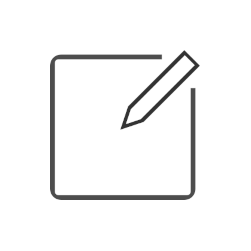# Drywall shapes order form

Whoops! Something went wrong.

##### L inside shape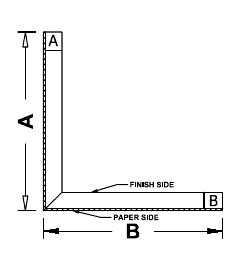• All dimensions must be listed in inches
• The total of A + B must = 48” or less
• A 54” board can be used if A + B = more than 48” and less than 54”
• Standard profile lengths are 96” (8’), 120” (10’), and 144” (12’)
• Custom profile lengths will incur additional cut charges
• Unless noted, all shapes will have 1 factory edge minimum
1.
Dimension A
Dimension B
Length
Board type
Thickness

Quantity
Comment
##### L outside shape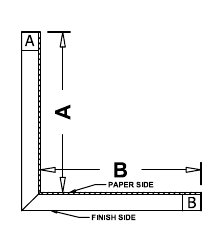• All dimensions must be listed in inches
• The total of A + B must = 46.75” or less
• A 54” board can be used if A + B = more than 46.75” and less than 52.75”
• Standard profile lengths are 96” (8’), 120” (10’), and 144” (12’)
• Custom profile lengths will incur additional cut charges
• Unless noted, all shapes will have 1 factory edge minimum
1.
Dimension A
Dimension B
Length
Board type
Thickness

Quantity
Comment
##### U inside shape• All dimensions must be listed in inches
• The total of A + B + C must = 48” or less
• A 54” board can be used if A + B = more than 48” and less than 54”
• Standard profile lengths are 96” (8’), 120” (10’), and 144” (12’)
• Custom profile lengths will incur additional cut charges
• Unless noted, all shapes will have 1 factory edge minimum
1.
Dimension A
Dimension B
Dimension C
Length
Board type
Thickness

Quantity
Comment
##### U outside shape1.
Dimension A
Dimension B
Dimension C
Length
Board type
Thickness

Quantity
Comment
##### Omega inside shape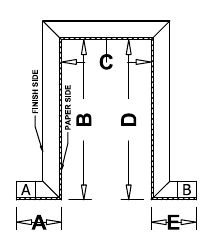• All dimensions must be listed in inches
• The total of A + B + C + D + E must = 45.5” or less
• A 54” board can be used if A + B + C + D + E = more than 45.5” and less than 51.5”
• Standard profile lengths are 96” (8’), 120” (10’), and 144” (12’)
• Custom profile lengths will incur additional cut charges
• Unless noted, all shapes will have 1 factory edge minimum
1.
Dimension A
Dimension B
Dimension C
Dimension D
Dimension E
Length
Board type
Thickness

Quantity
Comment
##### Omega outside shape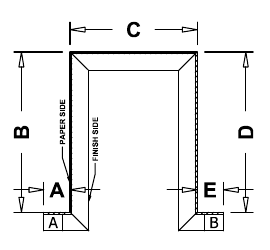• All dimensions must be listed in inches
• The total of A + B + C + D + E must = 45.5” or less
• A 54” board can be used if A + B + C + D + E = more than 45.5” and less than 51.5”
• Standard profile lengths are 96” (8’), 120” (10’), and 144” (12’)
• Custom profile lengths will incur additional cut charges
• Unless noted, all shapes will have 1 factory edge minimum
1.
Dimension A
Dimension B
Dimension C
Dimension D
Dimension E
Length
Board type
Thickness

Quantity
Comment
##### Z inside shape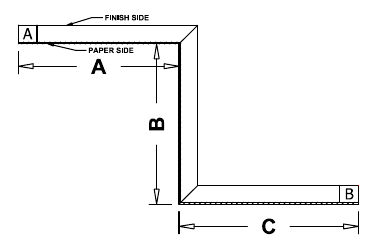• All dimensions must be listed in inches
• The total of A + B must = 46.75” or less
• A 54” board can be used if A + B = more than 46.75” and less than 52.75”
• Standard profile lengths are 96” (8’), 120” (10’), and 144” (12’)
• Custom profile lengths will incur additional cut charges
• Unless noted, all shapes will have 1 factory edge minimum
1.
Dimension A
Dimension B
Dimension C
Length
Board type
Thickness

Quantity
Comment
##### Z outside shape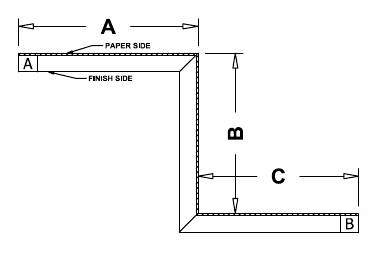• All dimensions must be listed in inches
• The total of A + B must = 46.75” or less
• A 54” board can be used if A + B = more than 46.75” and less than 52.75”
• Standard profile lengths are 96” (8’), 120” (10’), and 144” (12’)
• Custom profile lengths will incur additional cut charges
• Unless noted, all shapes will have 1 factory edge minimum
1.
Dimension A
Dimension B
Dimension C
Length
Board type
Thickness

Quantity
Comment
##### Step inside shape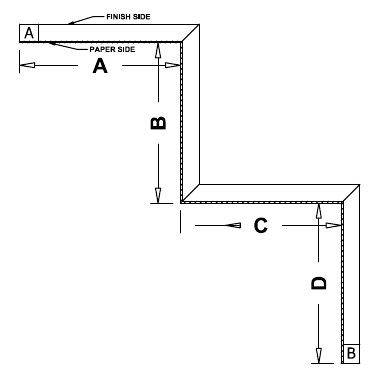• All dimensions must be listed in inches
• The total of A + B must = 46.75” or less
• A 54” board can be used if A + B = more than 46.75” and less than 52.75”
• Standard profile lengths are 96” (8’), 120” (10’), and 144” (12’)
• Custom profile lengths will incur additional cut charges
• Unless noted, all shapes will have 1 factory edge minimum
1.
Dimension A
Dimension B
Dimension C
Dimension D
Length
Board type
Thickness

Quantity
Comment
##### Step outside shape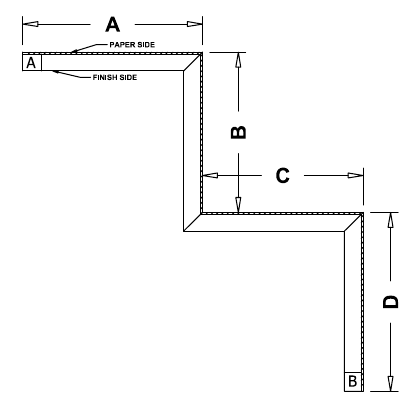• All dimensions must be listed in inches
• The total of A + B must = 45.5” or less
• A 54” board can be used if A + B = more than 45.5” and less than 51.5”
• Standard profile lengths are 96” (8’), 120” (10’), and 144” (12’)
• Custom profile lengths will incur additional cut charges
• Unless noted, all shapes will have 1 factory edge minimum
1.
Dimension A
Dimension B
Dimension C
Dimension D
Length
Board type
Thickness

Quantity
Comment
##### Drape pocket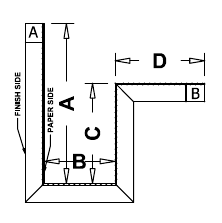• All dimensions must be listed in inches
• The total of A + B must = 45.5” or less
• A 54” board can be used if A + B = more than 45.5” and less than 51.5”
• Standard profile lengths are 96” (8’), 120” (10’), and 144” (12’)
• Custom profile lengths will incur additional cut charges
• Unless noted, all shapes will have 1 factory edge minimum
1.
Dimension A
Dimension B
Dimension C
Dimension D
Length
Board type
Thickness

Quantity
Comment
##### Light cove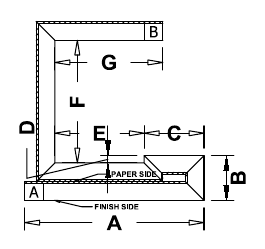• All dimensions must be listed in inches
• The total of A + B must = 42.75 or less
• A 54” board can be used if A + B = more than 42.75” and less than 48.75”
• Standard profile lengths are 96” (8’), 120” (10’), and 144” (12’)
• Custom profile lengths will incur additional cut charges
• Unless noted, all shapes will have 1 factory edge minimum
1.
Dimension A
Dimension B
Dimension C
Dimension D
Dimension E
Dimension F
Dimension G
Length
Board type
Thickness

Quantity
Comment
##### Window return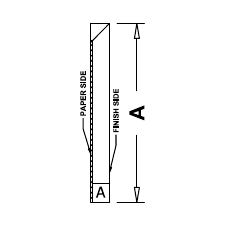• All dimensions must be listed in inches
• The total of A + B must = 47.325” or less
• A 54” board can be used if A + B = more than 47.325” and less than 53.325”
• Standard profile lengths are 96” (8’), 120” (10’), and 144” (12’)
• Custom profile lengths will incur additional cut charges
• Unless noted, all shapes will have 1 factory edge minimum
1.
Dimension A
Length
Board type
Thickness

Quantity
Comment
##### Castle cut• All dimensions must be listed in inches
• Standard profile lengths are 96” (8’), 120” (10’), and 144” (12’)
• Custom profile lengths will incur additional cut charges
• Unless noted, all shapes will have 1 factory edge minimum
1.
Dimension A
Dimension B
Dimension C
Dimension D
Dimension E
Dimension F
Length
Board type
Thickness
Quantity
Comment
##### Column wrap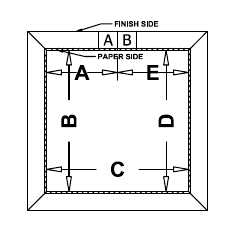• All dimensions must be listed in inches
• The total of A + B must = 48” or less
• A 54” board can be used if A + B = more than 48” and less than 54”
• Standard profile lengths are 96” (8’), 120” (10’), and 144” (12’)
• Custom profile lengths will incur additional cut charges
• Unless noted, all shapes will have 1 factory edge minimum
1.
Dimension A
Dimension B
Dimension C
Dimension D
Dimension E
Length
Board type
Thickness

Quantity
Comment
##### Ceiling tiles1.
Tile dimensions
Dimension A
Dimension B
Board type
Edge Profile
Grid Size
Style
Quantity
Comment